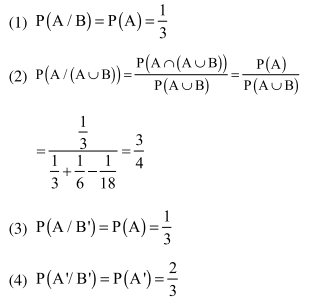Deepak Scored 45->99%ile with Bounce Back Crack Course. You can do it too!

# Solve this following

Question:

Let $\mathrm{A}$ and $\mathrm{B}$ be two independent events such that $P(A)=\frac{1}{3}$ and $P(B)=\frac{1}{6}$. Then, which of the following is TRUE?

1. $\mathrm{P}(\mathrm{A} / \mathrm{B})=\frac{2}{3}$

2. $\mathrm{P}(\mathrm{A} /(\mathrm{A} \cup \mathrm{B}))=\frac{1}{4}$

3. $\mathrm{P}\left(\mathrm{A} / \mathrm{B}^{\prime}\right)=\frac{1}{3}$

4. $P\left(A^{\prime} / B^{\prime}\right)=\frac{1}{3}$

Correct Option: , 3

Solution: